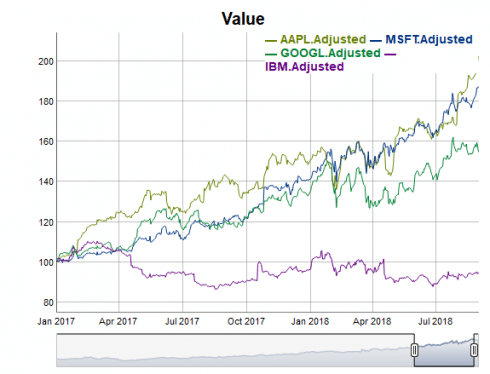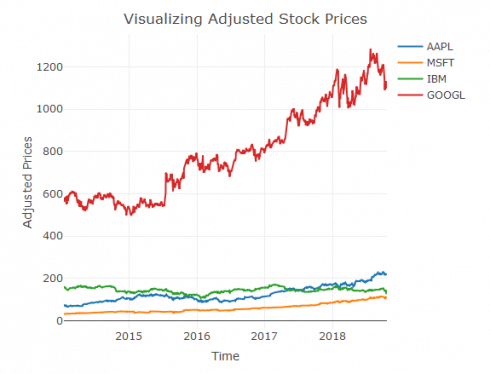Visualizations for Algorithmic Trading in R

June 3, 2019
By

(This article was first published on R Programming – DataScience+, and kindly contributed to R-bloggers)

Are you interested in guest posting? Publish at DataScience+ via your editor (i.e., RStudio).

Tags

Visualizations for Algorithmic trading is rising in demanded by the economic sector. In R there are a lot of great packages for getting data, visualizations and model strategies for algoritmic trading. In this article you learn how to perform visualizations and modelling for algorithmic trading in R

Algorithmic trading is a very popular machine learning method within the economic and financial sector. Typically it involves a lot of programming and visualization. The programming is nesecary in order to get the financial data for the Algorithmic Trading analysis.

First things first! We need to read these great packages into the R library:

```# Load R package
library(PortfolioEffectHFT)
library(rvest)
library(pbapply)
library(TTR)
library(dygraphs)
library(lubridate)
library(tidyquant)
library(timetk)
library(pacman)
library(quantmod)
library(parallelMap)
library(BiocParallel)
library(parallel)
library(plotly)
```

In the above packages you need to install `BiocParallel` with this code:

```## Install BiocParallel
source("https://bioconductor.org/biocLite.R")
biocLite("BiocParallel")
```

Next it is time to get the data. This involves a lot of programming in R:

```# Data Collection
table <- html_table(html_nodes(website, "table")[], fill = TRUE)
stocks.symbols<-table\$X2
stocks.names<-table\$X3
table1<-table[-1,-1]
colnames(table1)<-table[1,-1]
DT::datatable(table1)
stock.list<-"https://www.marketwatch.com/tools/industry/stocklist.asp?bcind_ind=9535&bcind_period=3mo"
stocks.names<-html_nodes(stocks,".lk01")
stocks.names<-html_text(stocks.names)
table1[table1==""] <- NA
table1<-table1[complete.cases(table1\$Symbol),]
DT::datatable(table1)
# Date creation
start.date<-Sys.Date()
end.date<-Sys.Date()-years(3)
start.date<-gsub('-','', start.date)
end.date<-gsub('-','', end.date)
start.date
# The symbols vector holds our tickers.
symbols <- c("SPY","EFA", "IJS", "EEM","AGG")
# The prices object will hold our raw price data
prices %
map(~Ad(get(.))) %>%   #Extract (transformed) data from a suitable OHLC object. getSymbols('IBM',src='yahoo') Ad(IBM)
reduce(merge) %>%   #reduce() combines from the left, reduce_right() combines from the right
`colnames<-`(symbols)
tickers <- c("AAPL", "MSFT","GOOGL","IBM","FB")
getSymbols(tickers)
closePrices <- do.call(merge, lapply(tickers, function(x) Cl(get(x))))
# Mapping
parallelStartSocket(2)
parallelStartMulticore(cpus=6)# start in socket mode and create 2 processes on localhost
f = function(x) Cl(get(x))     # define our job
y = parallelMap(f, tickers) # like R's Map but in parallel
# Bioconductor
options(MulticoreParam=quote(MulticoreParam(workers=4)))
param <- SnowParam(workers = 2, type = "SOCK")
vec=c(tickers,tickers,tickers,tickers)
multicoreParam <- MulticoreParam(workers = 7)
bio=bplapply(tickers, f, BPPARAM = multicoreParam)
```

Now we can make the first graph visualizations:

```# Visualization graph
dateWindow %
dyRebase(value = 100) %>%
dyRangeSelector(dateWindow = dateWindow)
end<-Sys.Date()
start<-Sys.Date()-years(3)
```

This gives us the following visualization graph with `dygraph`:Now loet us calculate the number of cores in the data and apply this in the visualization graph:

```# Calculate the number of cores
no_cores <- detectCores() - 1
# Initiate cluster
cl <- makeCluster(no_cores)
# vISUALIZATION inkl number of cores
dateWindow %
dyRebase(value = 100) %>%
dyRangeSelector(dateWindow = dateWindow)
```

This gives us the following visualization:Before making the third visualisation we need to do some more programming:

```# QUANTITATIVE MODELLING
getSymbols("AAPL",src='yahoo')
# basic example of ohlc charts
df <- data.frame(Date=index(AAPL),coredata(AAPL))
df <- tail(df, 30)
# cutom colors
i <- list(line = list(color = '#FFD700'))
d <- list(line = list(color = '#0000ff'))
p %
plot_ly(x = ~Date, type="ohlc",
open = ~AAPL.Open, close = ~AAPL.Close,
high = ~AAPL.High, low = ~AAPL.Low,
increasing = i, decreasing = d)
p
```

Next let us make a programmed visualization of adjusted stock prices over time:

```# Programmed visualization of adjusted stock prices over time
data%filter(Date>"2014-01-11")
p %
layout(title = "Visualizing Adjusted Stock Prices",
xaxis = list(title = "Time"),
yaxis = list (title = "Adjusted Prices"))
p
```

The above programming gives us the following visualization:Simple Trading Strategy with Trend Following

Next we will program a visualization of interactive Apple Moving Avereges over time:

```## Simple Trading Strategy: Trend Following
sdata%select(-Date)
#sdata<-tk_xts(data,date_var=Date)
df_50=as.data.frame.matrix(apply(sdata, 2, SMA,50))
colnames(df_50)=paste0(colnames(df_50),"_sma50")
df_200=as.data.frame.matrix(apply(sdata, 2, SMA,200))
colnames(df_200)=paste0(colnames(df_200),"_sma200")
df_all%drop_na()
# sma 50
f50<- function(x) SMA(x,50)
# sma 50
f200<- function(x) SMA(x,200)
dim(df_all)
# Moving avereges
mov.avgs<-function(df){

ifelse((nrow(df)<(2*260)),
x<-data.frame(df, 'NA', 'NA'),
x<-data.frame( SMA(df, 200), SMA(df, 50)))
colnames(x)<-c( 'sma_200','sma_50')
var=names(df_all)[str_detect(names(df_all), "AAPL")]
dateWindow=c("2015-01-01","2018-09-01")
dygraph(df_all[,var],main = 'Apple Moving Averages') %>%
dySeries('AAPL.Adjusted_sma50', label = 'sma 50') %>%
dySeries('AAPL.Adjusted_sma200', label = 'sma 200') %>%
dyRangeSelector(height = 30) %>%
dyShading(from = '2016-01-01', to = '2016-9-01', color = '#CCEBD6') %>%
dyShading(from = '2016-9-01', to = '2017-01-01', color = '#FFE6E6')%>%
dyRangeSelector(dateWindow = dateWindow)
```

Related Post

R-bloggers.com offers daily e-mail updates about R news and tutorials on topics such as: Data science, Big Data, R jobs, visualization (ggplot2, Boxplots, maps, animation), programming (RStudio, Sweave, LaTeX, SQL, Eclipse, git, hadoop, Web Scraping) statistics (regression, PCA, time series, trading) and more...